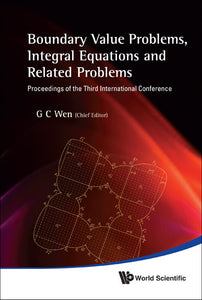# Boundary Value Problems, Integral Equations And Related Problems - Proceedings Of The Third International Conference

Guo Chun Wen

Format: Print Book

ISBN: 9789814327855

• SGD 230.05
Unit price per
Tax included.

In this volume, we report new results about various boundary value problems for partial differential equations and functional equations, theory and methods of integral equations and integral operators including singular integral equations, applications of boundary value problems and integral equations to mechanics and physics, numerical methods of integral equations and boundary value problems, theory and methods for inverse problems of mathematical physics, Clifford analysis and related problems.Contributors include: L Baratchart, B L Chen, D C Chen, S S Ding, K Q Lan, A Farajzadeh, M G Fei, T Kosztolowicz, A Makin, T Qian, J M Rassias, J Ryan, C-Q Ru, P Schiavone, P Wang, Q S Zhang, X Y Zhang, S Y Du, H Y Gao, X Li, Y Y Qiao, G C Wen, Z T Zhang, etc.

Format: Hardcover
No of Pages: 436
Imprint: World Scientific
Publication date: 20101223